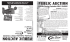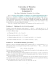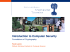# UOSEC Week 2: Asymmetric Cryptography

## Transcription

UOSEC Week 2: Asymmetric Cryptography
```UOSEC Week 2:
Asymmetric
Cryptography
Frank [email protected]
IRC kee
IRC xe0
IRC: irc.freenode.net #0x4f
Agenda
●
●
●
●
●
HackIM CTF Results
GITSC CTF this Saturday 10:00am
Basics of Asymmetric Encryption
Crypto Math
Implementation
Why Asymmetric vs Symmetric?
-Symmetric functions require both parties to share a secret
key to encrypt and decrypt messages, but transmitting a
secret key can be tricky.
-Asymmetric functions attempt to solve this issue by using
a private and public key pair.
Why Asymmetric vs Symmetric?
-Symmetric encryption follows the functions:
P = D(K,E(K,P))
-Asymmetric encryption looks like this:
P = D(KS, E(KP,P)), and P = D(KP, E(KS,P))
Where P= plaintext, K = key, KP = public key, KS = secret key, D() = decryption,
and E() = Encryption. Also, C = E(), where C = ciphertext
Why Asymmetric vs Symmetric?
-The asymmetric methods for encryption and decryption
mean that two different keys are needed, and only one
needs to be kept a secret (the decryption key).
-Encryption keys (or public keys) allow Alice and Bob to
send each other messages without risking their private
keys needed for decryption.
Public Keys
Alice needs Bob’s credit card information (She sells
scented candles) so she sends Bob her public key.
Alice
Alice’s KP
5f4dcc3b5aa765d61
d8327deb882cf99
Bob
Public Keys
Alice needs Bob’s credit card information (She sells
scented candles) so she sends Bob her public key.
Alice’s KP
Alice
Bob
5f4dcc3b5aa765d61
d8327deb882cf99
| Alice’s K
5f4dcc3b5aa765d61
Eve d8327deb882cf99
Eve is up to her old ways and catches Alice’s key.
P
Public Keys
Bob uses Alice’s public key to encrypt his card information.
E(KP, P) = C
Ciphertext
Alice
5ebe2294ecd0e0f08e
ab7690d2a6ee69
|
Eve
Alice’s KP
5f4dcc3b5aa765d61
d8327deb882cf99
Bob
Public Keys
Eve intercepts the ciphertext, and plans to decrypt it using
D(Kp, C).
Ciphertext
Alice
5ebe2294ecd0e0f08e
ab7690d2a6ee69
Ciphertext
5ebe2294ecd0e0f08e
ab7690d2a6ee69
|
Eve
Alice’s KP
5f4dcc3b5aa765d61
d8327deb882cf99
Bob
Public Keys
Alice uses her secret key KS to decrypt the ciphertext back
into plaintext, while Eve realizes something is not right...
Plaintext
Alice
Bob
“111 222 333 444
Visa, exp 1/19”
|
Eve
Plaintext
ae48aab730214004b
1f024d54757fccd
Eve learns that this may not be so easy.
Sending/Receiving Public Keys
-Send public keys as an email attachment, post them on
your web page, print out QR codes for your friends, etc.
-Public keys eliminate a great deal of issues when it comes
to sharing encryption keys.
-There are still some key management issues that cannot
be solved with public keys.
Key Management Issues
-How do we know that Alice’s public key really belongs to
Alice?
-If anyone can access a public key, how can one verify that
the sender is who they claim to be?
-The Web of Trust (WoT) and certificate authorities can
help.
Web of Trust
-Trust a third party entity to host public keys. (i.e. pgp.mit.
edu)
-Others can ‘digitally sign’ your public key to add credibility.
-The authenticity is only as good as the word of the people
backing it.
How Asymmetric Crypto Generally Works
-E() and D() are mathematical, one-way, inverse functions
of one another. (That is if E(x) = y, then D(y) = x.)
-By definition, a one-way function is a simple to compute
function, but extremely difficult to invert when given the
output. (E(x) = y is simple, but figuring out x when given y is
difficult.)
Just “difficult” to compute?
-Encryption algorithms are essentially really hard math
problems that would take a computer an unreasonably
large amount of time to determine. (Thousands of years in
some cases.)
-Based on Moore’s law, modern encryption will fail against
the computers of the future. Just like encryption methods of
the past fail today. (Try using WEP to protect your wireless
router some time.)
Prime Number Generation
-Using large prime numbers strengthens the cryptographic
equation, but how do we find such large prime numbers?
-Proving the Riemann Hypothesis would help (If you do
that, let me know.)
Checking for Primality
-For a number n, checking numbers 2 - √n for factors can
prove primality, but that will take forever.
-Are there other ways to check for primality?
Fermat’s Primality Test
Fermat came up with a test to determine if a number is
prime:
aP-1 = 1 mod P, where P = prime number, and 1 ≤ a < P.
Basically for any a,
aP-1 mod P = 1
Otherwise the number has factors.
Fermat’s Primality Test Examples
P=5
a4 mod 5 = 1
(1)4 : 1 mod 5 = 1 ✓
(2)4 : 16 mod 5 = 1 ✓
(3)4 : 81 mod 5 = 1 ✓
(4)4 : 256 mod 5 = 1 ✓
P=9
a8 mod 6 = 1
(1)8 = 1 mod 9 = 1 ✓
(2)8 = 256 mod 9 = 4 X
(3)8 = 6561 mod 9 = 0 X
(4)8 = 65536 mod 9 = 7 X
5 is prime.
9 is composite.
Exercise: Is this number prime?
563?
Exercise: Is this number prime?
563?
Yes!
Exercise: Is this number prime?
563?
Yes!
561?
Exercise: Is this number prime?
563?
Yes!
561?
It passes Fermat’s test, but 561 is not prime. Some
numbers can appear to be prime, but aren’t. (Carmichael
numbers)
Primality Tests
-Known tests can only give a probability of a number being
prime. These are less costly to perform than a factoring
algorithm.
-Miller Rabin Test, Fermat Primality Test
-Repeatedly testing a number can bring the likelihood of it
being prime into a high percentage (~99%)
Carmichael Numbers
-Carmichael numbers can pass Fermat’s primality test, but
are not actually prime.
-Using a non-prime number can severely reduce the
complexity of an encryption algorithm.
Discrete Log Problem
Consider 3x = 94143178827
Discrete Log Problem
Consider 3x = 94143178827
This can quickly be solved with ln(3x) = ln(94143178827)
x = 23
Discrete Log Problem
-Consider 3X mod 7 = 5, where x is an integer.
-Finding x would take a brute force attempt, which isn’t too
bad for mod 7, but consider:
12X mod 104729 = 100291
(12 is primitive root modulo 104729)
-The time complexity easily starts to get out of hand.
Some Asymmetric Implementations
-Diffie-Hellman Key Exchange (1976)
-RSA (Rivest, Shamir, Adelman 1977)
-ElGamal (1985)
-PGP (Pretty Good Privacy 1991)
Diffie-Hellman Key Exchange
-What if Alice and Bob can share a secret key without
-Provides a public means of key distribution.
-Allows an n participants to share a private key at one time.
Diffie-Hellman Algorithm
y = gx mod P
P = publicly agreed prime
g = a primitive root modulo P
x,y = either a public or private key (depending on the
phase)
Diffie-Hellman Algorithm
y = 5x mod 23
A public function is used. It
does not matter if this
function is intercepted.
Diffie-Hellman Algorithm
y = 5x mod 23
Alice: as = 6
Bob: bs = 15
Alice and Bob choose
secret numbers. Keys as
and bs are never shared.
Diffie-Hellman Algorithm
y = 5x mod 23
as = 6, bs = 15
Alice: ap = 56 mod 23 = 8
Bob: bp = 515 mod 23 = 19
Alice and Bob generate
their public keys
independent from one
another.
Once created, Alice will
share ap and Bob will share
b p.
Diffie-Hellman Algorithm
y = 5x mod 23
as = 6, bs = 15
ap = 8, bp = 19
Alice: SS = 196 mod 23 = 2
Bob: SS = 815 mod 23 = 2
The shared secret will be
computed separately by
both Alice and Bob when
they use each other’s public
in place of g.
2 will be their shared secret.
Who Knows What?
Alice
y = 5x mod 23
as = 6
ap = 8, bp = 19
SS = 2
Bob
y = 5x mod 23
bs = 15
ap = 8, bp = 19
SS = 2
Eve
y = 5x mod 23
ap = 8, bp = 19
Eve Is Left With Some Math Problems...
8 = 5a mod 23
19 = 5b mod 23
SS = 19a mod 23
SS = 8b mod 23
Look familiar?
The discrete log problem strikes again.
Diffie-Hellman Weaknesses
-New keys must be generated for every group of users.
-Cannot be used to sign certificates
-Issues when implemented in OpenSSL
RSA
-One public key can be shared with as many people as
needed.
-No need to manage multiple secret keys.
How RSA Works
Encryption: c = me mod n
Decryption: m = cd mod n
Where
n,e are the public key
d is the private key
m is plaintext, c is ciphertext
RSA Algorithm
-’Randomly’ generate two distinct prime numbers P and Q,
with similar bit length.
-n = PQ (This will be mod)
-Compute φ(n)
-Choose e such that e and φ(n) are coprime. (shorter bit
length is typically more efficient). e is the public key
exponent.
-Find d where de = 1 mod φ(n), d is the private key
exponent.
Coprime? φ(n)?
-Coprime numbers have a gcd of 1. (Example: 6 and 35)
-Euler's totient function φ(n) will give the amount of
numbers 1-n that are not factors of n.
φ(n) is also multiplicative. φ(j*k) = φ(j)*φ(k)
Examples:
φ(9) = 6 (That is 2,4,5,6,7,8)
φ(11) = 10 (Phi of a prime number φ(P) will always be P-1)
RSA Example
Step 1: Select primes P,Q
P = 61
Q = 53
Step 2: Compute n (PQ)
3233
RSA Example
Step 3: Find φ(n).
Since n = PQ, φ(PQ) = φ(P)*φ(Q) = φ(n).
φ(3233) = φ(61)*φ(53) = (60)(52) = 3120
Step 4: Find an e coprime with 3120, and 1< e <3120.
e = 17 (Smaller bit length is usually more efficient without
sacrificing complexity.)
RSA Example
Step 5: Find d where d(17) = 1 mod 3120. (The modular
multiplicative inverse)
d (17) mod 3120 = 1
2753(17) mod 3120 = 1
46801 mod 3120 = 1
d = 2753
RSA Example
We have our Encryption and Decryption functions!
Encryption: c = me mod n > c = m17 mod 3233
Decryption: m = cd mod n > m = c2753 mod 3233
Almost there...
Let’s encrypt an ascii “A” which is 65.
c = (65)17 mod 3233 = 2790
Almost there...
Finally, let’s decrypt 2790
m = (2790)2753 mod 3233 = 65
RSA Weaknesses
-Timing attacks (Listening to the CPU)
-Too small of an e compared to the modulus
-Chinese Remainder Theorem
Digital signatures in RSA
Message hash = h, Signature = s
Signing: h1d mod n = s
Verification: se mod n = h2
If h1= h2 the message is genuine and not tampered with.
Exercise: Brute Force RSA!
I secretly encoded a message using RSA. I want you to
decrypt it for me using only the following information:
e=5
N = 10142789312725007
c = 2010448083501228
helper files and copyable text at:
http://uosec.net/week2/
Exercise: Brute Force RSA!
e=5
N = 10142789312725007
c = 2010448083501228
hint: d*e ≡ 1 mod ɸ(N)
helper files and copyable text at:
http://uosec.net/week2/
Exercise: Brute Force RSA!
e=5
N = 10142789312725007
c = 2010448083501228
hint: d*e ≡ 1 mod ɸ(N)
hint2: m = cd mod N
helper files and copyable text at:
http://uosec.net/week2/
Exercise: Brute Force RSA!
Exercise: Brute Force RSA!
Steps to solve:
1. Factor 10142789312725007 using helper.py or wolfram
thus you get p = 100711409 q = 100711423
Exercise: Brute Force RSA!
Steps to solve:
1. Factor 10142789312725007 using helper.py or wolfram
thus you get p = 100711409 q = 100711423
2. Obtain decryption exponent d by solving
d = e-1 mod ɸ(N) = 8114231289041741
Exercise: Brute Force RSA!
Steps to solve:
1. Factor 10142789312725007 using helper.py or wolfram
thus you get p = 100711409 q = 100711423
2. Obtain decryption exponent d by solving
d = e-1 mod ɸ(N) = 8114231289041741
3. Get the message m = cd mod N = 8283659570766571
Exercise: Brute Force RSA!
Steps to solve:
1. Factor 10142789312725007 using helper.py or wolfram
thus you get p = 100711409 q = 100711423
2. Obtain decryption exponent d by solving
d = e-1 mod ɸ(N) = 8114231289041741
3. Get the message m = cd mod N = 8283659570766571
4. Break message into ascii aka. 82 = R, 83 = S, 65= A ...
Review
-Asymmetric cryptography relies on the Discrete Log
Problem.
-http://cryptopals.com/ has some exercises.
-Want to know more? Take a Cryptography class.
CIS 410 this Spring, or MATH 458 Spring ‘16.
```

### armedforces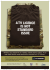### Homework 3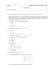### Semester I Examinations 2013-14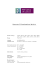### Untitled - Unfilodi### Discrete Math, Spring 2013 - Some Sample Problems### Lecture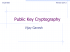### AUCTION INVENTORY REDUCTION &amp; MOVING Saturday, November 1 @ 10:00 AM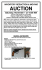### View Auction Flyer - Art Smith Auctioneers